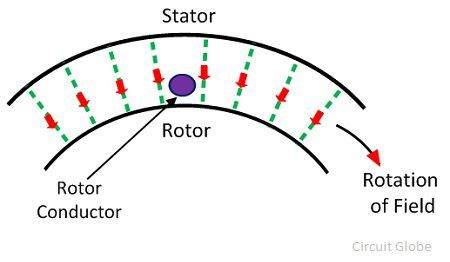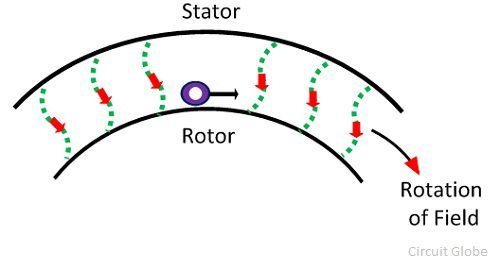# Working Principle of an Induction Motor

The motor which works on the principle of electromagnetic induction is known as the induction motor. Electromagnetic induction is the phenomenon in which the electromotive force induces across the electrical conductor when it is placed in a rotating magnetic field.

The stator and rotor are two essential parts of the motor. The stator is the stationary part, and it carries the overlapping windings while the rotor carries the main or field winding. The windings of the stator are equally displaced from each other by an angle of 120°.

The induction motor is the single excited motor, i.e., the supply is applied only to the one part, i.e., stator. The term excitation means the process of inducing the magnetic field on the parts of the motor.

When the three-phase supply is given to the stator, the rotating magnetic field produced on it. The figure below shows the rotating magnetic field set up in the stator:Consider that the rotating magnetic field induces in the anticlockwise direction. The rotating magnetic field has moving polarities. The polarities of the magnetic field vary by concerning the positive and negative half cycle of the supply. The change in polarities makes the magnetic field rotates.

The conductors of the rotor are stationary. This stationary conductor cut the rotating magnetic field of the stator, and because of the electromagnetic induction, the EMF induces in the rotor. This EMF is known as the rotor induced EMF, and it is because of the electromagnetic induction phenomenon.

The conductors of the rotor are short-circuited either by the end rings or by the help of the external resistance. The relative motion between the rotating magnetic field and the rotor conductor induces the current in the rotor conductors. As the current flows through the conductor, the flux induces on it. The direction of rotor flux is the same as that of the rotor current.

Now we have two fluxes one because of the rotor and another because of the stator. These fluxes interact with each other. On one end of the conductor the fluxes cancel each other, and on the other end, the density of the flux is very high. Thus, the high-density flux tries to push the conductor of the rotor towards the low-density flux region. This phenomenon induces the torque on the conductor, and this torque is known as electromagnetic torque.

The direction of electromagnetic torque and the rotating magnetic field is the same. Thus, the rotor starts rotating in the same direction as that of the rotating magnetic field.

The speed of the rotor is always less than the rotating magnetic field or synchronous speed. The rotor tries to run at the speed of the rotor, but it always slips away. Thus, the motor never runs at the speed of the rotating magnetic field, and this is the reason because of which the induction motor is also known as the asynchronous motor.

### Why Rotor never runs at Synchronous Speed?

If the speed of the rotor is equal to the synchronous speed, no relative motion occurs between the rotating magnetic field of the stator and the conductors of the rotor. Thus the EMF is not induced on the conductor, and zero current develops on it. Without current, the torque is also not produced.

Because of the above mention reasons the rotor never rotates at a synchronous speed. The speed of the rotor is always less than the speed of the rotating magnetic field.

Alternatively, the method of the working principle of the Induction Motor can also be explained as follows.

Let’s understand this by considering the single conductor on the stationary rotor. This conductor cuts the rotating magnetic field of the stator. Consider that the rotating magnetic field rotates in the clockwise direction. According to Faraday’s Law of electromagnetic induction, the EMF induces in the conductor.As the rotor circuit is completed by the external resistance or by the end ring, the rotor induces an EMF which causes the current in the circuit. The direction of the rotor induces current is opposite to that of the rotating magnetic field. The rotor current induces the flux in the rotor. The direction of the rotor flux is the same as that of the current.The interaction of rotor and stator fluxes develops a force that acts on the conductors of the rotor. The force acts tangentially on the rotor and hence induces torque. The torque pushes the conductors of the rotor, and thus the rotor starts moving in the direction of the rotating magnetic field. The rotor starts moving without any additional excitation system and because of this reason the motor is called the self-starting motor.The operation of the motor depends on the voltage induced on the rotor, and hence it is called the induction motor.

### 18 thoughts on “Working Principle of an Induction Motor”

1. I would say the best website to provide such detailed content. Please do provide for other topics as well.